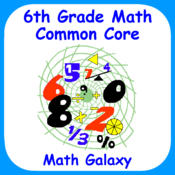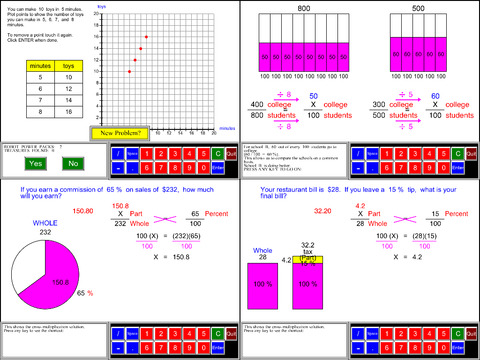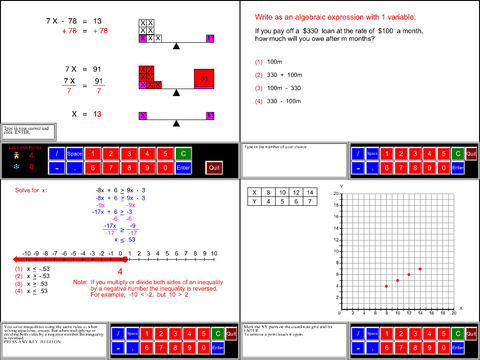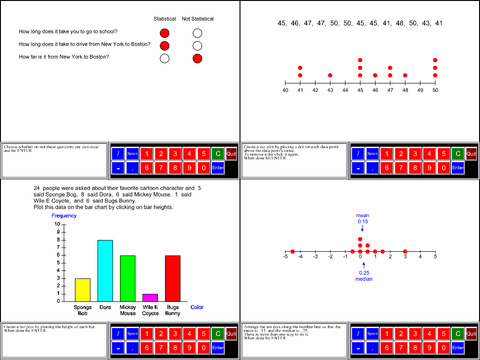# 6th Grade Math Common Core - Lumos Educational App Store41
Price -\$5.99
\$5.99

#### DESCRIPTION:

Math Galaxy's Sixth Grade Common Core Math covers all of the Common Core math standards for sixth grade as listed below.The concepts are presented in bite-size pieces with an unlimited supply of practice problems, with numbers chosen randomly.Illustrations are used throughout to reinforce the concepts.By solving problems students earn robots to use in a maze game.Certificates with scores can be emailed to be printed.RATIOS and PROPORTIONAL RELATIONSHIPS (6.RP)Ratios & Proportions Understanding Ratios (6.RP.1) Rates (6.RP.2) Ratios in Tables

#### OVERVIEW:

6th Grade Math Common Core is a free educational mobile app By Math Galaxy.It helps students in grades 6 practice the following standards 6.EE.1,6.EE.2,6.EE.9.

This page not only allows students and teachers download 6th Grade Math Common Core but also find engaging Sample Questions, Videos, Pins, Worksheets, Books related to the following topics.

1. 6.EE.1 : Write and evaluate numerical expressions involving whole-number exponents..

2. 6.EE.2 : Write, read, and evaluate expressions in which letters stand for numbers..

3. 6.EE.9 : Use variables to represent two quantities in a real-world problem that change in relationship to one another; write an equation to express one quantity, thought of as the dependent variable, in terms of the other quantity, thought of as the independent variable. Analyze the relationship between the dependent and independent variables using graphs and tables, and relate these to the equation. For example, in a problem involving motion at constant speed, list and graph ordered pairs of distances and times, and write the equation d = 65t to represent the relationship between distance and time..

6

#### STANDARDS:

6.EE.1
6.EE.2
6.EE.9
6.G.1
6.G.2
6.G.3
6.G.4
6.NS.1
6.NS.2
6.NS.3
6.NS.4
6.NS.5
6.NS.6
6.NS.7
6.NS.8
6.RP.1
6.RP.2
6.RP.A.3
6.SP.1
6.SP.2
6.SP.3
6.SP.4
6.SP.5

Developer: Math Galaxy

Software Version: 1.2

Category: Education

Release Date: July 21,2015### RELATED APPSEdSearch WebSearch